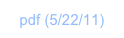Dissecting “FibTriangle”Overview

In this tutorial, we look at the inner workings of the fibtriangle program, introduced in The Fib Triangle, and also at FibTri from the Demos folder.

fibtriangle is written in RPL+. It takes a number off the stack, and computes the Fibonacci numbers from 1 to the given number. Every number computed is right-aligned and added as a row to an image. As the Fibonacci numbers get greater and greater, the resulting image takes on a triangular shape.

The program doesn’t utilize the built-in fib (Classic: FIB) function to produce each Fibonacci number, but instead uses a very simple stack operation to produce each successive Fibonacci number. 400 Fibonacci numbers create a 400-row image, that is, it’s one number per row.

fibtriangle

You can edit or visit the code for fibtriangle. It looks like this:

0: ≪ → n ≪

1:    "FibTriangle"

2:    ‘ceil(n*0.7/8)*2’ EVAL =width

3:    1 1 →big toHex

4:    "" =s

5:      1 n START

6:        @dup @rotate +

7:        @dup @toString 3 0 substring width "0" pad

8:        s @swap + =s

9:      NEXT

10:    DROP2

11:    "0x" @swap +

12:    width 4 *

13:    n

14:    image

≫≫≫

Here’s what it does, line by line:

Line 0 stores the input number into variable “n”

Line 1 puts the name of the result image on the stack

Line 2 determines the width of the image that will result

Line 3 puts two 1s on the stack and converts the second one into a BigNum integer, and hexadecimal display

Line 4 pushes an empty string into a local variable s; this is the beginning of our image data, which will be added row by row with the loop that follows next

Line 5 begins a loop from 1 to n; in this loop, the following steps are performed:

Line 6     computes the next Fibonacci number using the contents of stack positions 1 and 2

Line 7     wraps that number in a string,

strips the “0x” at the beginning of the number, and pads the result with 0 to achieve a length of width

Line 8     prepends the resulting string (which is now a full hexadecimal row of the image) to s

(which is the image data string / accumulation of equal-length image rows so far)

Line 9 close the loop

Line 10 deletes the two top-most stack positions, which were used as intermediate results

Line 11 prepends “0x” to the image data string to make it look like a hexadecimal number wraps in a string

Line 12 pushes the width the desired image on the stack; for the desired binary image there are 4 pixels (4 bits)

in each hexadecimal digit, hence the computed width is multiplied by four

Line 13 pushes the height of the desired image on the stack

Line 14 constructs the image from the name, data, and width and height arguments which are now on the stack

Note, this RPL program uses the “modern” naming of functions.

In Classic mode, toHex is →hex, @toString is →STR, size is SIZE, sub is SUB, and @swap is SWAP. The functionality is identical. The Classic names match HP calculators. The intention for modern naming is easier to type and read code.FibTri

You can edit or visit the code for FibTri in the Demos folder. It looks like this:

function(n) {

var width = ceil(n*0.7/8)*2;

var data = "0x";

var a = BigInteger.ZERO; var one = BigInteger.ONE; var b = one;

for (var i=0; i<n; i++) {

var val = BigNum["+"](a, b);

a = b; b = val;

// convert number to hex, string, slice off 0x, pad w/ zeroes, and concatenate with data string

}

return NDImage.toImage(string.toString("FibTri" + n), string.toString(data), width*4, n);

}

The function works in pretty much the same way.

You find copies of the RPL+ and JavaScript functions in the BigInt folder, with timing code added: fibtr_rpl and fibtr_JS. Run these on the number 400, for example, to compare timings. You’ll notice that there’s only a small speed advantage for JavaScript. That’s because in both implementations most of the time is spent in BigNum arithmetic, which runs at the same speed in both languages.